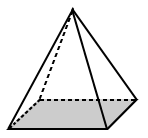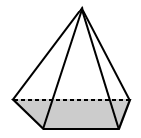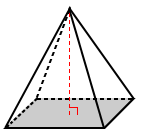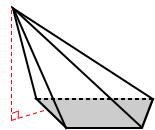# Pyramid

In Geometry, a pyramid is a space figure that has a polygon as its base and triangles as all its other faces. All the faces of a pyramid, except its base, intersect at a common point, called the apex. The following are some examples.

Outside of geometry, the term "pyramid" is often used in reference to the pyramids of Egypt, which served as tombs for ancient Egyptian kings. Pyramids are sometimes used in modern construction, as in the Louvre, a famous museum in Paris.

## What is a pyramid

A pyramid is a three-dimensional (3D) figure with triangular faces attached to a polygonal base. The base may be a square, triangle, or some other polygon.

### Parts of a pyramid

The main parts that make up a pyramid are its base, faces, and apex.

• Base: The base of the pyramid is the face of the pyramid that all of the lateral faces are attached to. It is the only face of the pyramid that does not intersect the apex.

• Face: One of the polygonal surfaces of a pyramid.

• Lateral face: The faces of a pyramid not including the base.

• Apex: The top of the pyramid where all of its lateral faces meet.

Note that if the base of a given pyramid is a regular polygon, the lateral faces are congruent isosceles triangles.

#### Pyramid - Faces, edges, vertices

Pyramids, like other polyhedrons, also have edges and vertices. The number of faces, edges, and vertices of a pyramid is dependent on the base of a pyramid. For example, a square pyramid has 4 lateral faces and 1 square base for a total of 5 faces; 8 edges; 5 vertices. Note that a pyramid will always have the same number of faces and vertices. The formulas for finding the faces, edges, and vertices of a pyramid are as follows:

• Faces: n + 1

• Vertices: n + 1

• Edges: 2n

where n is the number of sides of the base of the pyramid.

## Pyramid properties

The faces of a pyramid that are not its base are called lateral faces. The number of lateral faces in a pyramid is the same as the number of sides in its polygonal base. The line segments created by two intersecting faces are called edges. The vertices are points where three or more edges meet. The vertex opposite the base is called its apex. The apex is often thought of as the "top" of the pyramid. It is also the common point shared by all the lateral faces of the pyramid.

The square pyramid above has a total of five faces. It has 4 lateral faces that are triangles and its base is a square. A square pyramid has 8 edges and 5 vertices.

Any cross section that is parallel to the base of a pyramid forms a polygon that is similar to the base.

The three triangles, shaded in green, formed by cross sections parallel to the base of the triangular pyramid above, are similar (same shape but not the same size) to the pyramid's base.

## Types of pyramids

A pyramid is named based on the shape of its polygonal base. The following are some examples.

### Regular pyramid

A regular pyramid is a pyramid in which the base is a regular polygon. Often, a regular pyramid is implied to be a right pyramid.A square is a regular polygon, so the square pyramid above is a regular pyramid.

### Irregular pyramid

An irregular pyramid is a pyramid in which the base is not a regular polygon. An example of an irregular pyramid is a trapezoidal pyramid:### Right pyramid

A right pyramid is a pyramid in which the apex is directly above the center of the base. In a right pyramid, a perpendicular line drawn from the apex will intersect the base at its center.### Oblique pyramid

An oblique pyramid is a pyramid in which the apex is not directly above the center of the base. In an oblique pyramid, a perpendicular line drawn from the apex does not intersect the base at its center.### Tetrahedron

A tetrahedron is a special pyramid whose faces are all triangles, including the base. It is the only type of pyramid in which any of its faces can be the base and any of its vertices can be the apex.

## Formula for pyramid

The formulas for the surface area and volume of a pyramid are described below.

### Volume of a pyramid

The volume of pyramid is ⅓ the area of the base multiplied by the height of the pyramid:B is the area of the base and h is the height of the pyramid, which is the distance from its apex to its base.

The formula is dependent on the type of polygonal base. For example, the area of the base of the rectangular pyramid on the left is A = length × width. The area of the trapezoidal pyramid on the right is, where a and b are the lengths of the bases and h is the height.

### Surface area of a pyramid

The surface area of a pyramid is dependent on the base of the pyramid. The total surface area is the sum of the area of the base and the sum of the areas of the lateral faces. Find the areas of each and the sum of these areas to find the total surface area of the pyramid.